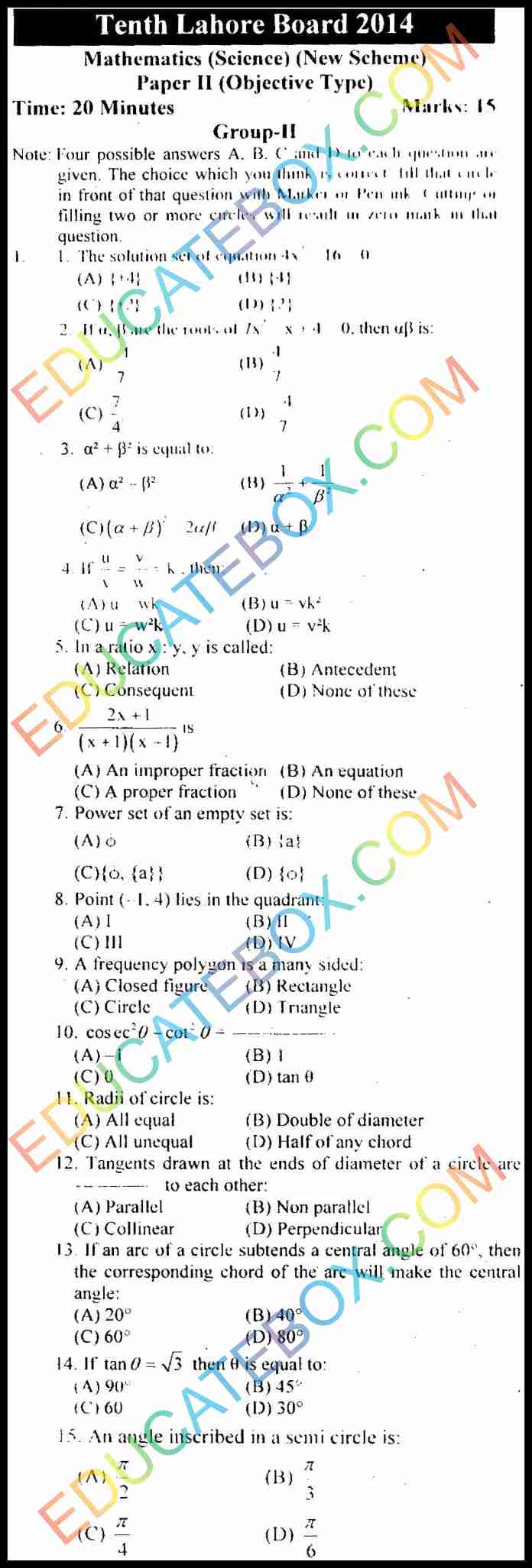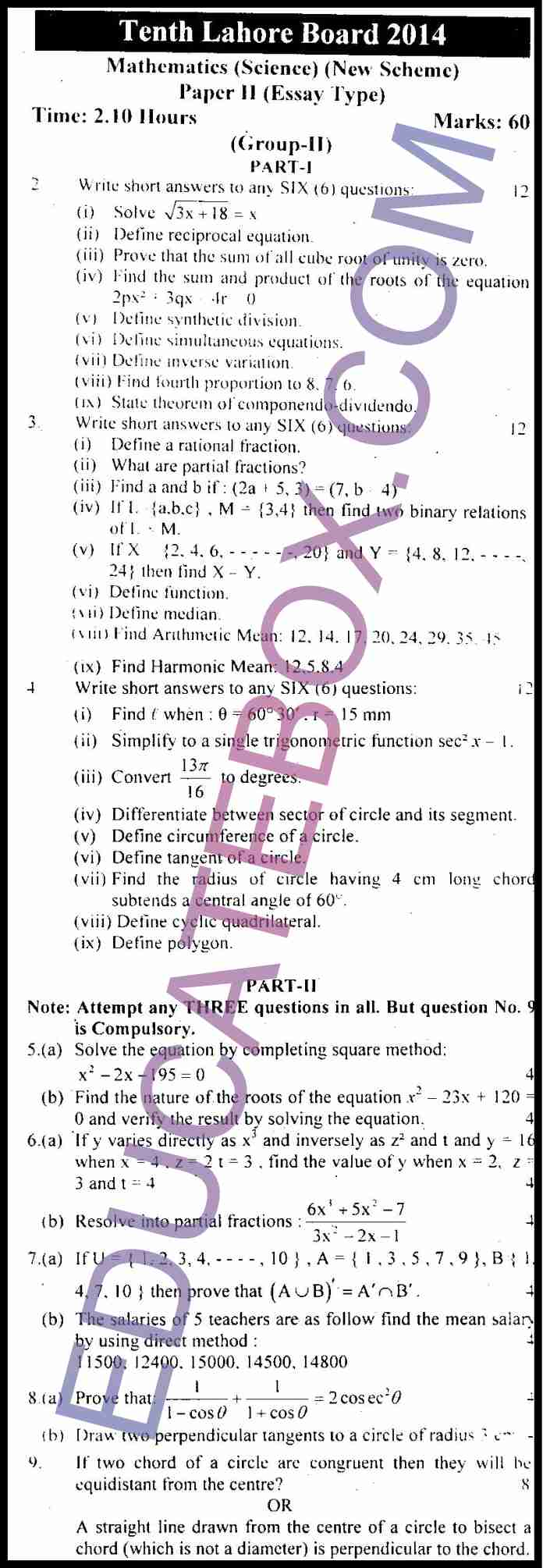# Past Paper 10th Class Maths (Science Group) Lahore Board 2014 Group 2

0
338

## Past Paper Class 10 Maths (Science Group) Lahore Board 2014 Objective Type Group 2 (English Medium)

Here is past paper of class 10 Maths (Science Group) Lahore Board 2014 objective type paper of Group 2 (English Medium).   In the MCQ part, four possible answers A, B, C and D to each question are given. The choice which you think is correct, fill that circle in front of that question with Marker or Pen ink. Cutting or filling two or more circles will result in zero mark in that question.

Some of the questions in multiple choice questions portion are as follows:

• The solution set of equation 4X2 – 16 = 0
• What is Power set of an empty set?
• Point (-1, 4 ) lies in the quadrant?
• If an arc of a circle subtends a central angle of 60s, then the corresponding chord of the arc will make the central angle?
• What is an angle inscribed in semi-circle?## Past Paper Class 10 Maths (Science Group) Lahore Board 2014 Subjective Type Group 2 (English Medium)

Subjective portion consists of long questions and short questions. Some of them are as follows:

• Define reciprocal equation
• Prove that the sum of all cube root of unity is zero.
• Find fourth proportion to 8, 7, 6
• Find the radius of circle having 4 cm long chord subtends a central angle of 60.
• If two chord of a circle are congruent then they will be equidistant from the centre?
• A straight line drawn from the centre of a circle to bisect a chord (which is not a diameter) is perpendicular to the chord.